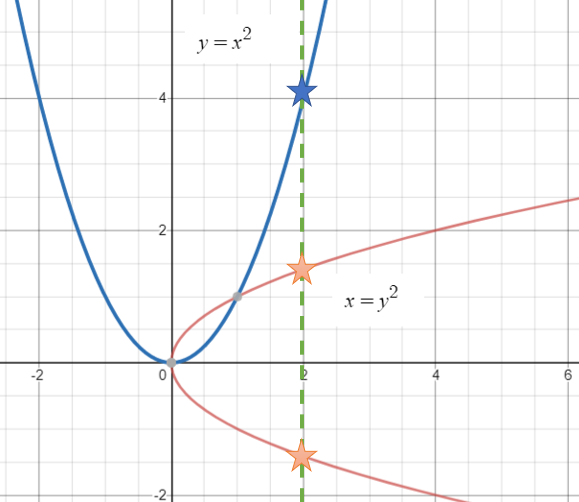# Formula vs Function

Functions > Formula vs Function

Contents:

## Formula vs Function: What is a Formula?

A formula is a mathematical sentence with at least one variable. For example 2 + 4 isn’t a formula (there’s no variable), but 2x + 4 is a formula, because there’s a variable. Formulas are used in math to describe relationships. Variables can be any letter from a to z, but you’ll want to follow some general rules for writing formulae:

• Abbreviate variables to one letter. For example, if you have a variable for weight, shorten that to w, not we or wt. This is because when you have two variables in a formula it means to multiply them, so “wt” means “w * t”.
• Use small letters a, b, c, … z.
• Omit the multiplication sign (unless you’re multiplying two numbers like 2*4). Write 2x, not 2 * x.
• Put the number first if you’re multiplying a variable by a number. Write 2x, not x2.

## Formula vs Function: Functions

In general math and beginning algebra, you work with formulas. But in calculus, you work with functions. The difference between formulas and functions is in how they are constructed: functions are a specific type of formula with exactly one output (y) for every input (x).

• For example, if you put a “2” into the formula y = x2, there’s only one output: 4. That’s a function.
• If you put a 2 into the formula x = y2, there are two possibilities for each input (x-value): 8 and -8.

Let’s take a look at these graphically:At x = 2, the blue function y = x2 has one output (blue star), but the formula y = x2 has two outputs (orange stars).

All functions are formulas, but not all formulas are functions.

## Formula vs Function in Excel

A “function” in Excel is a predefined formula. All Excel built-in functions are also functions in the traditional sense (i.e. you’ll get one output for every input).

As an example, let’s say you wanted to add a string of numbers from 1 to 4. All formulas in Excel start with an equals sign “=”. So you might type the formula “=1+2+3+4”. You could also invoke the built in function SUM to do the same thing: =SUM(1,2,3,4).

When making small calculations like this, there isn’t much of a difference between a formula and a function in Excel. Where the big difference comes in is when you’re dealing with large amounts of data in cells (something which Excel was designed for). If you had 1,000 numbers in column A, it might take some time to write the formula with all the numbers from 1 to 1,000:
=1+2+3+4+5+6…1,000
But you can type in a function that will add up all of the numbers between cells A1 and cells A2 in seconds:
SUM(A1:A1000).

## References

Graph created with Desmos.com.

CITE THIS AS:
Stephanie Glen. "Formula vs Function" From StatisticsHowTo.com: Elementary Statistics for the rest of us! https://www.statisticshowto.com/formula-vs-function/

### 2 thoughts on “Formula vs Function”

1. Hi Stephanie, thanks for putting together a great resource here, I may even pick up your book ;)
I’m just writing to you here as I think I have spotted a typo on your types of functions page: https://www.statisticshowto.com/types-of-functions/

I couldn’t leave a reply on that particular page so I’m writing it to you here. Anyway at the top of that page it has: “1. What is a Function?
A function is a type of equation or formula that has exactly one output (y) for every input (x). If you put a “2” into the equation x2, there’s only one output: 4. Some formulas, like x = y2, are not types of functions, because there are two possibilities for each x-value (one positive and one negative).”

I think you meant to put there are two possibilities for each y value (2 and -2), instead of each x-value.

Kind regards,
Michael.

2. Thank you very much for letting me know, Michael. It is fixed.